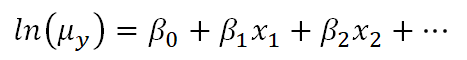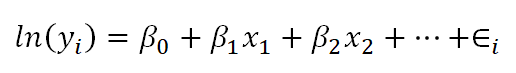# The Difference Between Link Functions and Data Transformations

Generalized linear models—and generalized linear mixed models—are called generalized linear because they connect a model’s outcome to its predictors in a linear way. The function used to make this connection is called a link function. Link functions sounds like an exotic term, but they’re actually much simpler than they sound.

For example, Poisson regression (commonly used for outcomes that are counts) makes use of a natural log link function as follows:Clearly, there is not a direct linear relationship of the x variables to the average count, but there is a “sort of linear” relationship happening: a function of the mean of y is related to a linear combination of x variables. In other words, the linear model has now been generalized to a bigger type of situation.

This can lead to confusion, though, because on the surface it looks very similar to what happens when we transform the dependent variable in a linear model, like a linear regression.

The key thing to understand is that the natural log link function is a function of the mean of y, not the y values themselves.

### Transformations of Y

Below is a linear model equation where the original dependent variable, y, has been natural log transformed. That is, the natural log has been taken of each individual value of y, and that is being used as the dependent variable.The linear model with the log transformation is providing an equation for an individual value of ln(y). We could also write it as follows, where we are modeling the mean of ln(y) (note the error term is no longer present):This makes the difference a bit clearer. When we transform the data in a linear model, we are no longer claiming that y is normally distributed around a mean, given the x values — we are claiming that our new outcome variable, ln(yi), is normally distributed.

In fact, we often make this transformation specifically because the values of y do not appear to be normally distributed around their average.

In the case of the Poisson model, however, the link function does not change the distribution of the actual observations in some way to make them something other than Poisson distributed. Instead, the link function defines the relationship of the x variables directly to the mean of the Poisson distributed y. The individual observations then vary around this expected value accordingly.

### The mean of the log is not the log of the mean

As you may know, if you have used this kind of data transformation in a linear model before, you cannot simply take the exponent of the mean of ln(y) to get the mean of y.

You might be surprised to know, though, that you can do this with a link function. If you have specific values of your x variables, you can calculate the predicted average count, μy based on those x values by inversing the natural log:This ability to back-transform means (and regression coefficients) to a more intuitive scale is part of what makes generalized linear models so useful.

#### Go to the next article or see the full series on Easy-to-Confuse Statistical ConceptsWhat is GLMM and When Should You Use It?
When you have multilevel or repeated data and normality just isn't happening, you may need GLMM. Get started learning Generalized Linear Mixed Models and when and how to apply them to your data.

### Related Posts

1.Alan Mainwaring says

I have been grappling with the Generalized Linear Model, The General Linear Model for years. Students just coming out of High School have real problems even with the term “Linear”.
First I had to throw out the idea that Linearity applied to the [predictor variables only. The focus for linearity is on the Parameters, another difficult idea.
This posting on explaining the difference between a function of a single dependent value for some fixed value of a predictor value and that of a function of the mean of now y being a random variable and thus associated with a probability distribution is a real step forward as to the understanding of what a link function is.
Thank you very much for this clearly explained difference

2.Prasanth peddaayyavarla says

You guys rock!!!!
Thank you.

Please note that, due to the large number of comments submitted, any questions on problems related to a personal study/project will not be answered. We suggest joining Statistically Speaking, where you have access to a private forum and more resources 24/7.